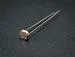Published

# Cracking an electronic safe using brute force

I couldn't find the code to my safe and needed to retrieve documents from it, so I cracked it.

Full instructions provided10,238## Things used in this project

### Hardware components

 Fireproof safe
×1
 Relay (generic)
×1Photo resistor
×1Jumper wires (generic)
×1Arduino UNO
×1

Read more

## Code

### arduino_sketch_safe_cracker.c

C/C++
arduino_sketch_safe_cracker.c
```/*
* Brute force safe cracker for a Sentry Fire Safe.  It took me 5 days
* to open my safe with this.  YMMV.  Have fun!
*/

// map of keypad key number to arduino pin controlling the corresponding relay

unsigned char key2pin = {
9, // kp0
2, // kp1
6, // kp2
10,// kp3
3, // kp4
7, // kp5
11,// kp6
4, // kp7
8, // kp8
12 // kp9
};

// arduino pin mapped to power cycling relay

unsigned char powerpin = 5;

int verbose = true;

// arduino pins mapped to photocells on green and red LEDs

int grnPin = 0;
int redPin = 1;

void setup()
{
Serial.begin(9600);

// Initialize key relays and power relay to open (high is open)
int i;
for(i = 0; i <= 9; i++)
{
digitalWrite(key2pin[i],HIGH);
pinMode(key2pin[i],OUTPUT);
}
digitalWrite(powerpin, HIGH);
pinMode(powerpin, OUTPUT);
}

// Read the status LEDs.  Return either the number of red blinks (the
// usual case), or that we got a green LED, which indicates a cracked
// safe.

int readStatus(double time, int& redBlink)
{
// These values should be independently calibrated
int redThresh = 200;
int grnThresh = 200;

int nsamples = 1000*time;

redBlink = 0;
int grnBlink = 0;

// Count blinks via a moving avg and threshold cross

int len = 10; // moving average length

double avgRed = 0.0;
double avgGrn = 0.0;
double avgRed_last = 0.0;
double avgGrn_last = 0.0;

double redVals[len];
for (int n = 0; n < len; n++) {
redVals[n] = 0.0;
}

double grnVals[len];
for (int n = 0; n < len; n++) {
grnVals[n] = 0.0;
}

for (int n = 0; n < nsamples; n++) {

// shift values to free up position 0
for (int k = 9; k >= 1; k--) {
redVals[k] = redVals[k-1];
grnVals[k] = grnVals[k-1];
}
redVals = analogRead(redPin);
grnVals = analogRead(grnPin);

double sumRed = 0;
for (int k = 0; k < len; k++) {
sumRed += redVals[k];
}
double avgRed = sumRed / len;

double sumGrn = 0.0;
for (int k = 0; k < 10; k++) {
sumGrn += grnVals[k];
}
double avgGrn = sumGrn / len;

if (avgRed > redThresh && avgRed_last < redThresh) {
redBlink++;
}
avgRed_last = avgRed;

if (avgGrn > grnThresh && avgGrn_last < grnThresh) {
grnBlink++;
}
avgGrn_last = avgGrn;

delay(time/nsamples*1e3);
}

return grnBlink;
}

void turn_on()
{
if (verbose) Serial.println("turning on");
digitalWrite(powerpin,LOW);
}

void long_power_cycle()
{
if (verbose) Serial.println("long power cycle");
digitalWrite(powerpin, HIGH);
delay(5000);
digitalWrite(powerpin, LOW);
delay(400);
}

void power_cycle()
{
if (verbose) Serial.print("power cycle... ");
digitalWrite(powerpin,HIGH);
delay(3250);
digitalWrite(powerpin,LOW);
delay(400);
if (verbose) Serial.println(" done.");
}

void press(int key)
{
digitalWrite(key2pin[key], LOW);  // CLOSE
delay(100);
digitalWrite(key2pin[key], HIGH); // OPEN
delay(250);
}

// try all combinations of buttons we can press

void loop()
{
int lo = 0;
int hi = 9;

int attempt_count = 0;

int cracked = 0;

turn_on();
delay(500);

for (int digit1 = lo; digit1 <= hi; digit1++) {
for (int digit2 = lo; digit2 <= hi; digit2++) {
for (int digit3 = lo; digit3 <= hi; digit3++) {
for (int digit4 = lo; digit4 <= hi; digit4++) {
for (int digit5 = lo; digit5 <= hi; digit5++) {

attempt_count++;

int redBlinks = 0;

if (verbose) Serial.print("Trying ");
Serial.print(digit1);
Serial.print(digit2);
Serial.print(digit3);
Serial.print(digit4);
Serial.print(digit5);
if (verbose) Serial.println();

press(digit1);
press(digit2);
press(digit3);
press(digit4);
press(digit5);

cracked = readStatus(2.0, redBlinks);

// 3 red blinks is a wrong combination.  5 red
// blinks is some mysterious error code.  When we
// get one of those, do a long power cycle to try to
// get things back to normal.

if (redBlinks > 3) long_power_cycle();

Serial.print(redBlinks);
if (verbose) Serial.println(" red blinks");

Serial.print(cracked);
if (verbose) Serial.println(" green blinks");

if (cracked) {
Serial.print("Cracked!  Code is ");
Serial.print(digit1);
Serial.print(digit2);
Serial.print(digit3);
Serial.print(digit4);
Serial.print(digit5);
while (1) {
}
}

// We need to power cycle after 3 failed attempts to get
// out of lockout mode

if (attempt_count == 3) {
power_cycle();
attempt_count = 0;
}

}
}
}
}
}
}
```

## Credits

### Bob Anderson

1 project • 3 followers
I like solving problems, especially DIY.